Ex 8.1

Chapter 8 Class 10 Introduction to Trignometry
Serial order wise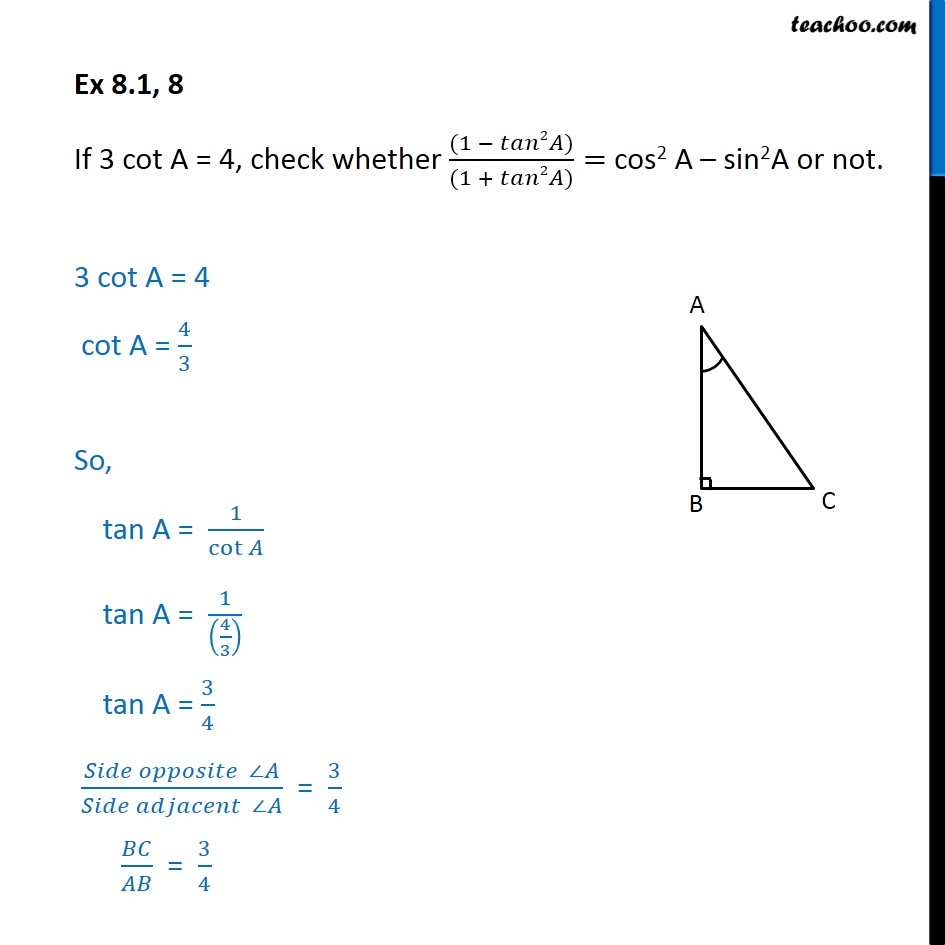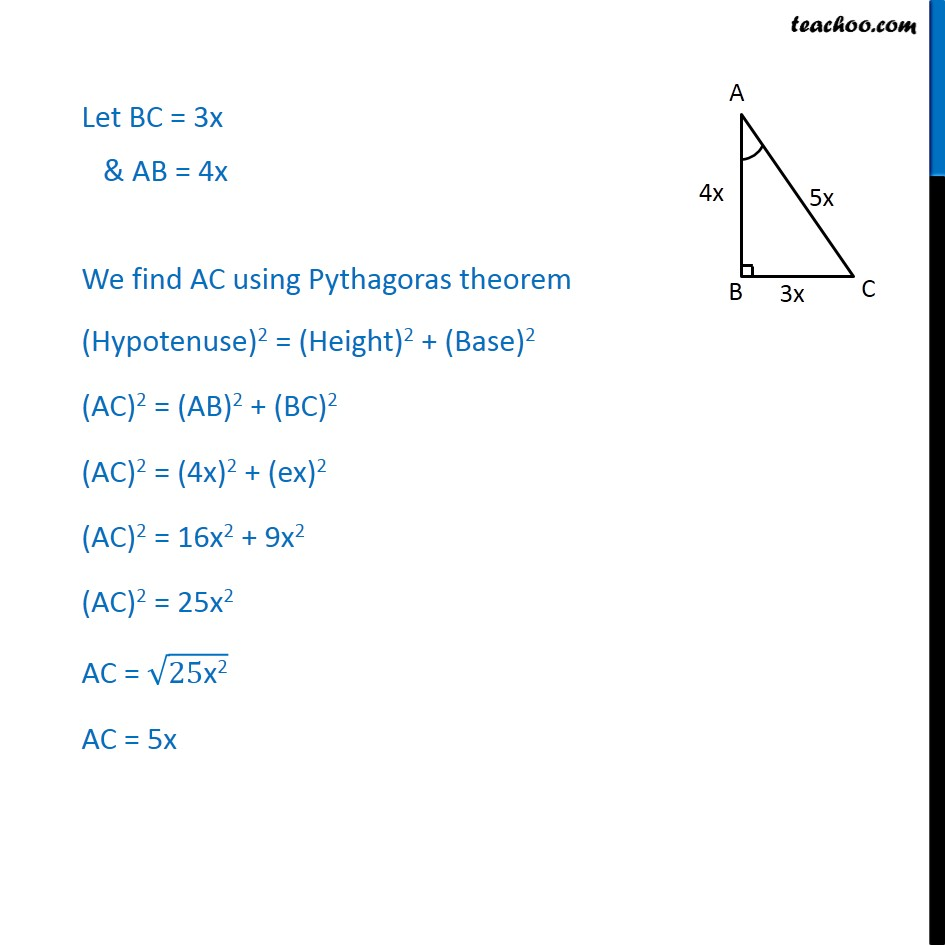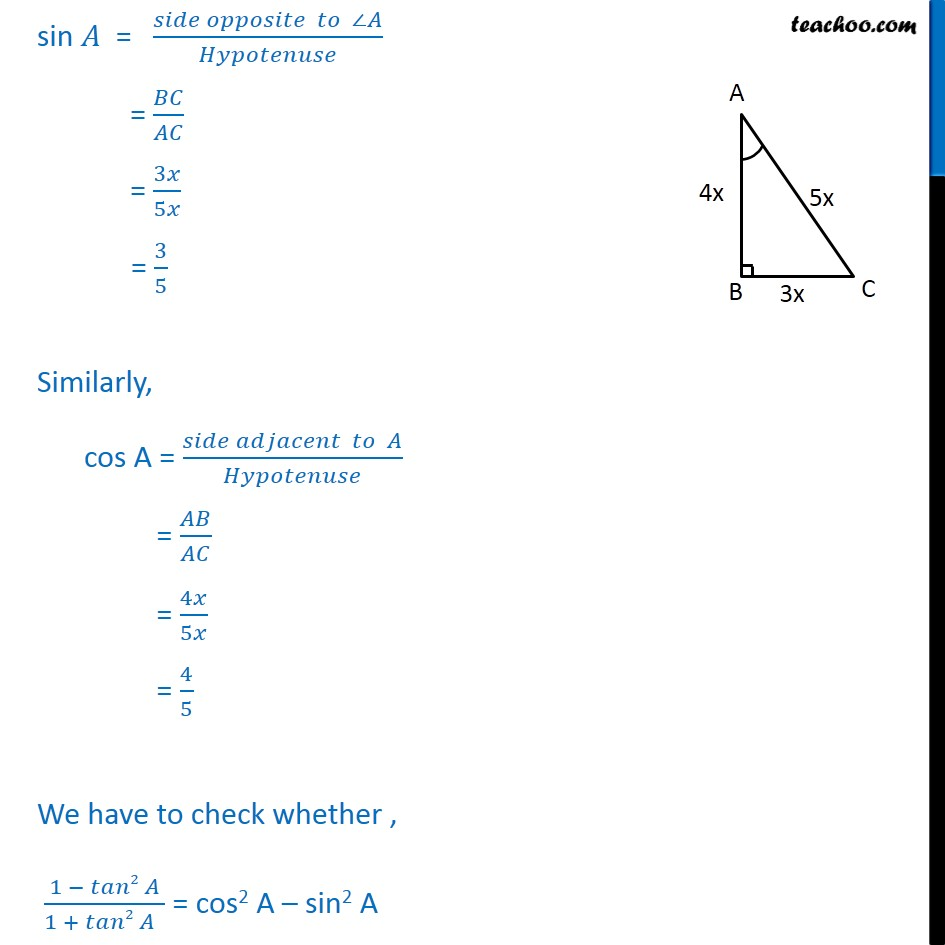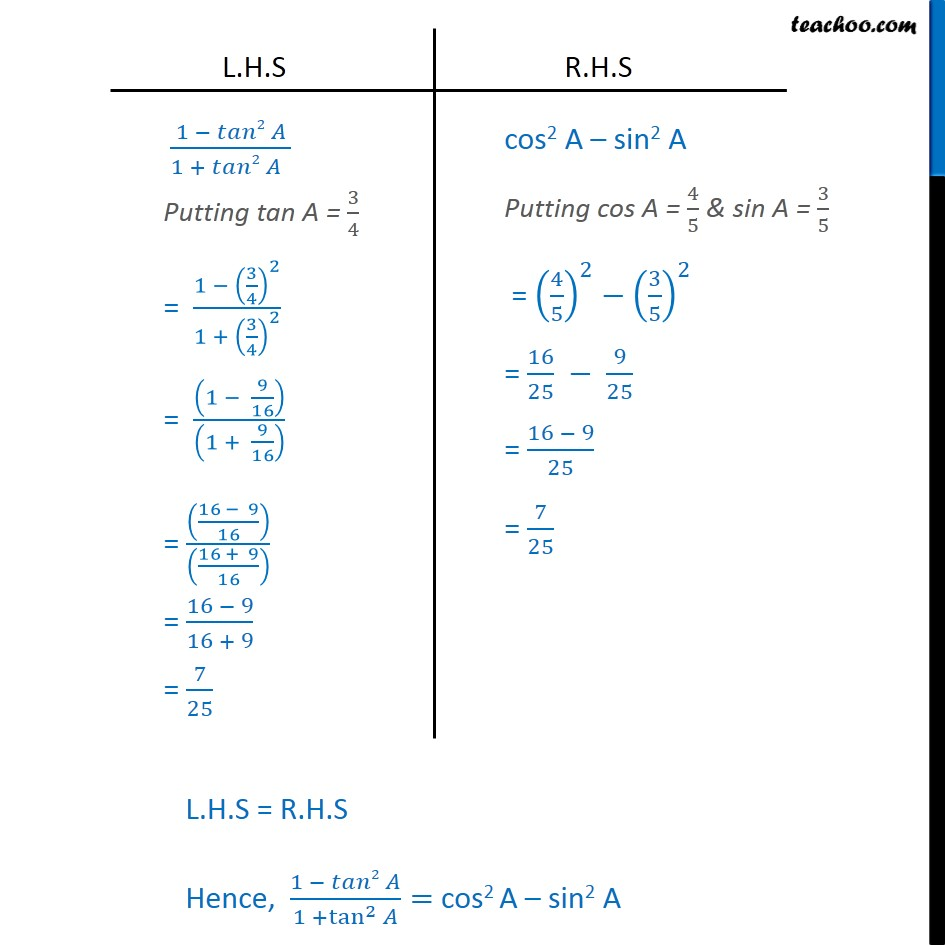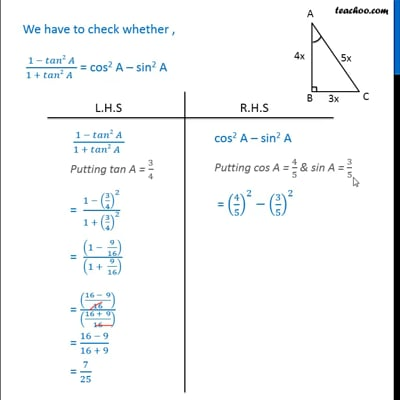This video is only available for Teachoo black users

Get live Maths 1-on-1 Classs - Class 6 to 12

### Transcript

Ex 8.1, 8 If 3 cot A = 4, check whether ((1 − 𝑡𝑎𝑛2𝐴))/((1 + 𝑡𝑎𝑛2𝐴))= cos2 A – sin2A or not. 3 cot A = 4 cot A = 4/3 So, tan A = 1/cot⁡𝐴 tan A = 1/((4/3) ) tan A = 3/4 (𝑆𝑖𝑑𝑒 𝑜𝑝𝑝𝑜𝑠𝑖𝑡𝑒 ∠𝐴)/(𝑆𝑖𝑑𝑒 𝑎𝑑𝑗𝑎𝑐𝑒𝑛𝑡 ∠𝐴) = 3/4 𝐵𝐶/𝐴𝐵 = 3/4 Let BC = 3x & AB = 4x We find AC using Pythagoras theorem (Hypotenuse)2 = (Height)2 + (Base)2 (AC)2 = (AB)2 + (BC)2 (AC)2 = (4x)2 + (ex)2 (AC)2 = 16x2 + 9x2 (AC)2 = 25x2 AC = √(25"x2" ) AC = 5x sin 𝐴 = (𝑠𝑖𝑑𝑒 𝑜𝑝𝑝𝑜𝑠𝑖𝑡𝑒 𝑡𝑜 ∠𝐴)/𝐻𝑦𝑝𝑜𝑡𝑒𝑛𝑢𝑠𝑒 = 𝐵𝐶/𝐴𝐶 = 3𝑥/5𝑥 = 3/5 Similarly, cos A = (𝑠𝑖𝑑𝑒 𝑎𝑑𝑗𝑎𝑐𝑒𝑛𝑡 𝑡𝑜 𝐴)/𝐻𝑦𝑝𝑜𝑡𝑒𝑛𝑢𝑠𝑒 = 𝐴𝐵/𝐴𝐶 = 4𝑥/5𝑥 = 4/5 We have to check whether , (1 − 𝑡𝑎𝑛2 𝐴)/(1 + 𝑡𝑎𝑛2 𝐴 ) = cos2 A – sin2 A